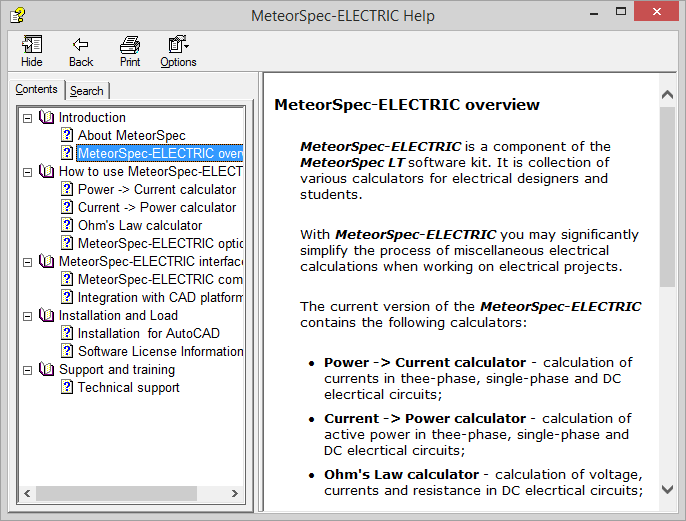## MeteorSpec-ELECTRIC

MeteorSpec-ELECTRIC - collection of calculators for electricians.

### Features summary

MeteorSpec-ELECTRIC is a CAD plugin that contains a collection of various calculators for electrical designers and students. MeteorSpec-ELECTRIC version 2 provides the following calculations:

• Current carrying capacity calculator - calculation of continuous current-carrying capacity of multi-core power cables;
• Power -> Current calculator - calculation of currents in three-phase, single-phase and DC electrical circuits;
• Current -> Power calculator - calculation of active power in three-phase, single-phase and DC electrical circuits;
• Ohm's Law calculator - calculation of voltage, currents and resistance in DC electrical circuits.

Units of measurement used in the calculations can be customized over a wide range. The MeteorSpec-ELECTRIC also allows you to flexibly adjust the accuracy of the result values.

### Current carrying capacity calculator

Using this command you can calculate the continuous current carrying capacity of multicore cables in three-phase or single-phase AC electrical circuits. The calculation procedure is based on the IEC 60364-5-52 standard.

The user can define various methods of installation and ambient properties. Also determination of the current carrying capacity is carried out taking into account influence of grouped cables.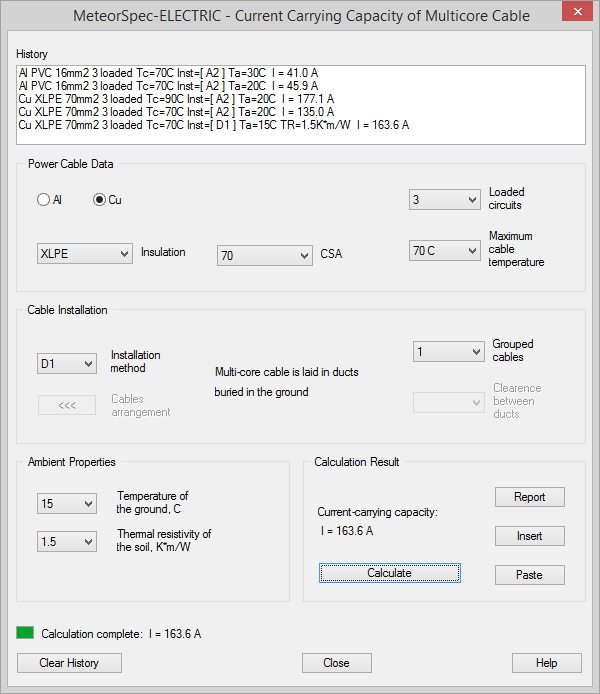Click on the "Report" button to view the full report of the calculation procedure.

The calculator supports only cable constructions that are covered by the reference standard. The calculator has the following features:

• The calculator is intended for calculations of current carrying capacity of low-voltage multi-core cables only.
• The current version of the software supports the single-layer arrangement of grouped cables only.
• The depth of cables laying underground is 0.7m.

### Power -> Current calculator

Using this calculator you can calculate currents in three-phase or single-phase AC electrical circuits, if the value of active power is known. Also you can calculate direct currents in DC electrical circuits, if the value of power is known.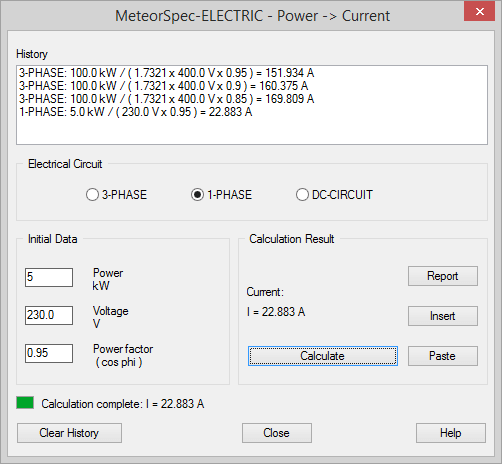Click on the "Report" button to to view the full report of the calculation procedure.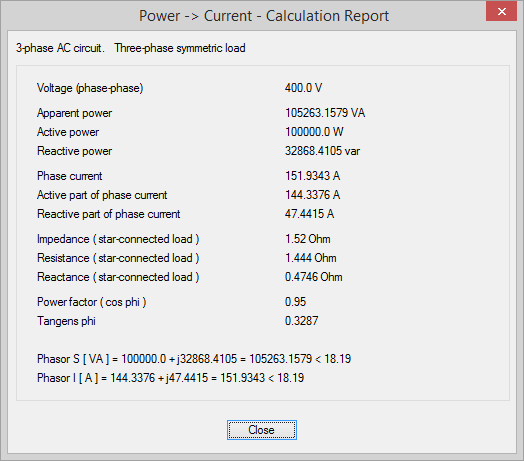### Current -> Power calculator

Using this calculator you can calculate active power in three-phase or single-phase AC electrical circuits, if the value of current (rms) is known. Also you can calculate power in DC electrical circuits, if the value of direct current is known.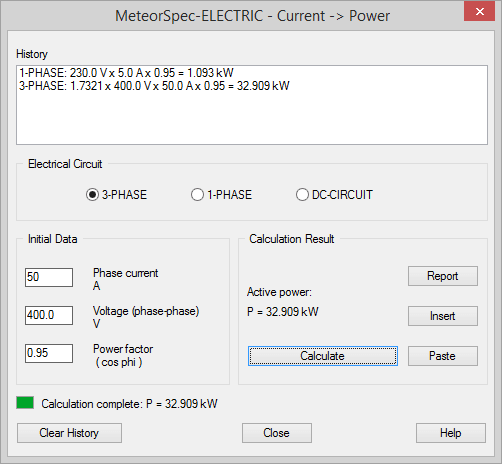### Ohm's Law calculator

Using this calculator you can calculate voltage, currents and resistance in DC electrical circuits.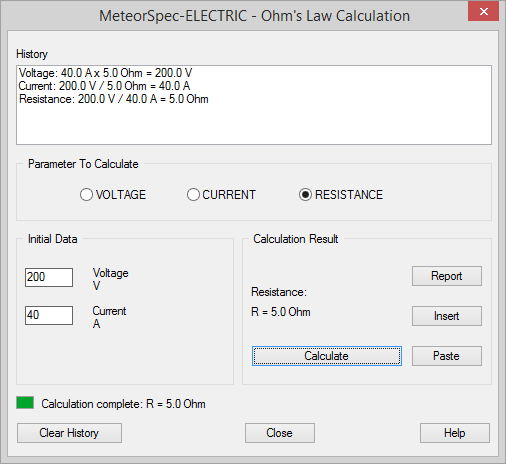### Help System

The build-in Help system describes the operation of MeteorSpec-ELECTRIC.